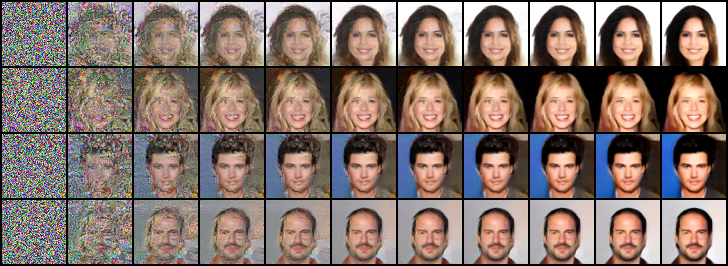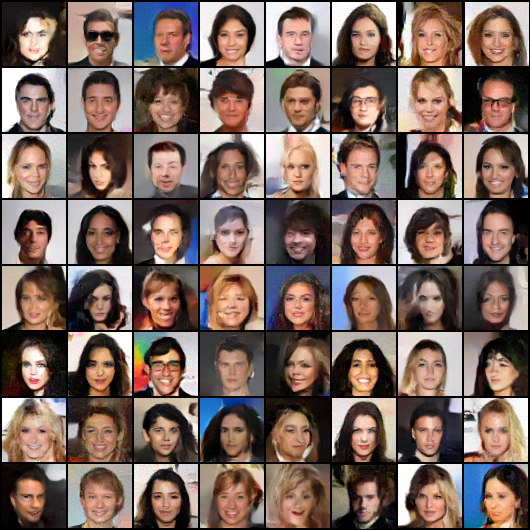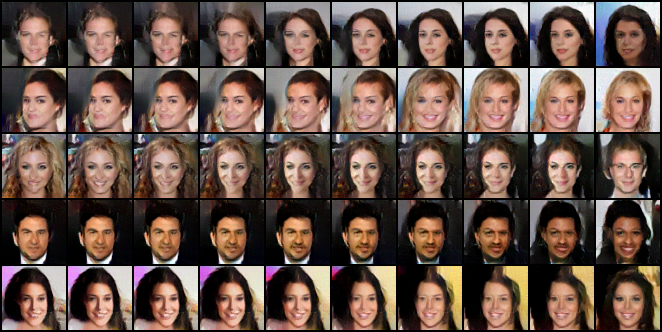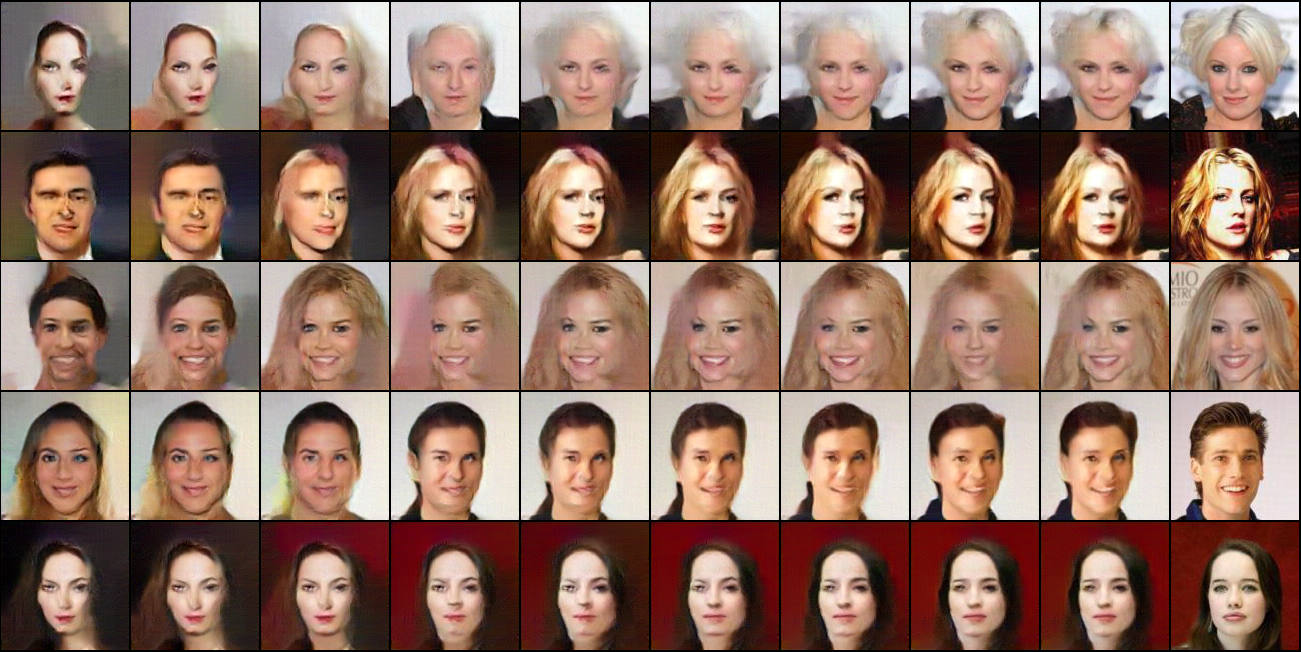# Learning Non-Convergent Non-Persistent Short-Run MCMC Toward Energy-Based Model

University of California, Los Angeles (UCLA), USA

## Abstract

This paper studies a curious phenomenon in learning energy-based model (EBM) using MCMC. In each learning iteration, we generate synthesized examples by running a non-convergent, non-mixing, and non-persistent short-run MCMC toward the current model, always starting from the same initial distribution such as uniform noise distribution, and always running a fixed number of MCMC steps. After generating synthesized examples, we then update the model parameters according to the maximum likelihood learning gradient, as if the synthesized examples are fair samples from the current model. We treat this non-convergent short-run MCMC as a learned generator model or a flow model. We provide arguments for treating the learned non-convergent short-run MCMC as a valid model. We show that the learned short-run MCMC is capable of generating realistic images. More interestingly, unlike traditional EBM or MCMC, the learned short-run MCMC is capable of reconstructing observed images and interpolating between images, like generator or flow models.

## Paper

The publication can be obtained here.
@article{nijkamp2019shortrun,
title={Learning Non-Convergent Non-Persistent Short-Run MCMC Toward Energy-Based Model},
author={Nijkamp, Erik and Hill, Mitch and Zhu, Song-Chun and Wu, Ying Nian},
journal={NeurIPS 2019},
year={2019}
}


## Poster

The poster can be obtained here.

## Code

The code can be obtained here.

## Experiments

### Experiment 1: Synthesis

We evaluate the fidelity of generated examples on various datasets, each reduced to $$40,000$$ observed examples. Figure 1 depicts the transition of short-run MCMC from uniform noise towards realistic images. Figure 2 depicts generated samples for various datasets with $$K=100$$ Langevin steps for both training and evaluation. Despite its simplicity, short-run MCMC is competitive.Figure 1: Generating synthesized examples by running 100 steps of Langevin dynamics initialized from uniform noise for CelebA $$(64\times64)$$.Figure 2: Generated samples for K = 100 MCMC steps. From left to right: (1) CIFAR-10 ($$32 \times 32$$), (2) CelebA ($$64 \times 64)$$, (3) LSUN Bedroom ($$64 \times 64)$$.

### Experiment 2: Interpolation

We show that unlike traditional EBM and MCMC, the learned short-run MCMC is capable of interpolating different images, just like a generator or a flow model can do. Figure 3 depicts short-run MCMC with with interpolated noise $$z_\rho = \rho z_1 + \sqrt{1-\rho^2} z_2$$ where $$\rho\in[0,1]$$ on CelebA $$(64\times64)$$.Figure 3: Interpolation by short-run MCMC resembling a generator model: The transition depicts the sequence $$M_\theta(z_\rho)$$ with interpolated noise $$z_\rho = \rho z_1 + \sqrt{1-\rho^2} z_2$$ where $$\rho\in[0,1]$$ on CelebA ($$64 \times 64)$$. Left: $$M_\theta(z_1)$$. Right: $$M_\theta(z_2)$$.

### Experiment 3: Reconstruction

We demonstrate reconstruction of observed examples. For an observed image $$x$$, we reconstruct $$x$$ by running gradient descent on the least squares loss function $$L(z) = \|x - M_\theta(z)\|^2$$, initializing from $$z_0 \sim p_0(z)$$, and iterates $$z_{t+1} = z_{t} - \eta_t L'(z_t)$$. The reconstruction ability of the short-run MCMC is due to the fact that it is not mixing.Figure 4: Reconstruction by short-run MCMC resembling a generator or flow model: The transition depicts $$M_\theta(z_t)$$ over time $$t$$ from random initialization $$t=0$$ to reconstruction $$t=200$$ on CelebA ($$64 \times 64$$). Left: Random initialization. Right: Observed examples.

## Acknowledgements

The work is supported by DARPA XAI project N66001-17-2-4029; ARO project W911NF1810296; and ONR MURI project N00014-16-1-2007; and XSEDE grant ASC170063. We thank Prof. Stu Geman, Prof. Xianfeng (David) Gu, Diederik P. Kingma, Guodong Zhang, and Will Grathwohl for helpful discussions.